# Conditional Rendering in VueJS

Learn about the Conditional Rendering in VueJS.
Submitted by Venkatesan C, on March 13, 2022

## Conditional Rendering

• It is very common in the front-end applications, where you have to hide and show certain elements based on some information. Let's say you want to hide the navigation bar, if the user is not logged in. In such cases, we have to conditionally render the elements.
• VueJs helps us to conditionally render elements based on values of properties or expressions.

Let's look at an example to understand it better:

Create a Vue project using the Vite build tool. Let's name it second-app (in fact this is the same project that's been created in the VueJS installation tutorial).

Here is the project structure: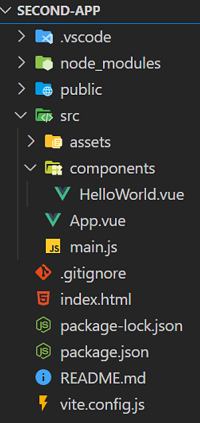Now delete everything inside the App.vue file and paste the below code. The below is simpler to understand the binding inline styles in VueJs concept.

App.vue

```<template>
<h1 v-if="number == 0">Number is equal to 0</h1>
<h1 v-else-if="number < 0">Number is less than 0</h1>
<h1 v-else-if="number > 0">Number is greater than 0</h1>
<h1 v-else>This is not a number</h1>

<div v-if="canShow">
<h4>Introduction</h4>
the concept of conditional rendering.</p>
</div>
<template v-if="canShow">
<h4>Contact info</h4>
<p>[email protected].com</p>
<p>+1 528-624-85</p>
<h1 v-if="canShow">Just a demo using v-if</h1>
<h1 v-show="canShow">Just a demo using v-show</h1>
</template>
</template>

<script>
export default {
name: "App",
data() {
return {
number: -9,
canShow: true
};
},
};
</script>
```

We can conditionally render elements in VueJS by using the following directives,

• v-if
• v-else-if
• v-else
• v-show

In the App.vue file, we have four <h1> tags to show how v-if, v-else-if, and v-else directives help us to conditionally render elements based on the value of the expression.

• If the number is equal to zero, "Number is equal to 0" is rendered on to the DOM,
• Or if the number is less than zero, "Number is less than 0" will be rendered,
• Or if the number is greater than zero, "Number is greater than 0" will be rendered,
• Else "This is not a number" will be rendered.

## Conditionally rendering a section of elements

• Let's say you want to render a group of Html elements, to render in such a way, you have to wrap the HTML elements under a <div> tag, then conditionally render the div tag using v-if on it,
• But here, there is an additional <div> tag that will be rendered onto the DOM, which is unnecessary,
• Therefore, Vue provides the <template> tag to avoid such unnecessary elements getting added to the DOM tree,
• <template> will not get rendered onto the DOM.

### v-show vs v-if

• v-show also conditionally renders the element as how v-if works, but with one difference that v-show conditionally renders the element by manipulating the display property to none,
• Whereas v-if removes the element from the DOM itself, if the condition evaluates to false.

Output:

For the following data values, "Number is less than 0" is displayed. And both the introduction and contact info sections are displayed.

``` number: -9,
canShow: true
```

Try changing the value of the number by yourself to get better understanding.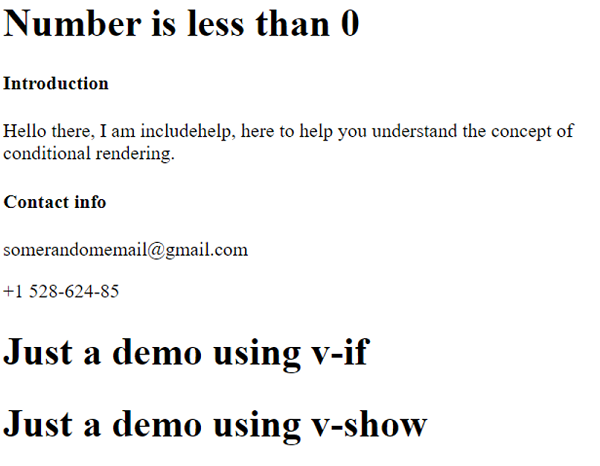Inspecting the element shows that the <template> tag doesn't get rendered whereas the <div> got rendered onto the DOM,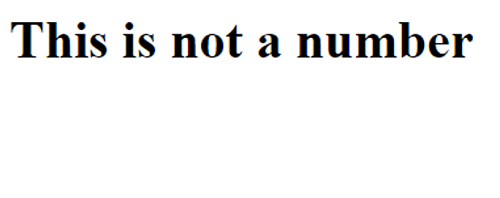Let's change the values of the data, as follows and look at the output,

``` number: "I am a string",
canShow: false
```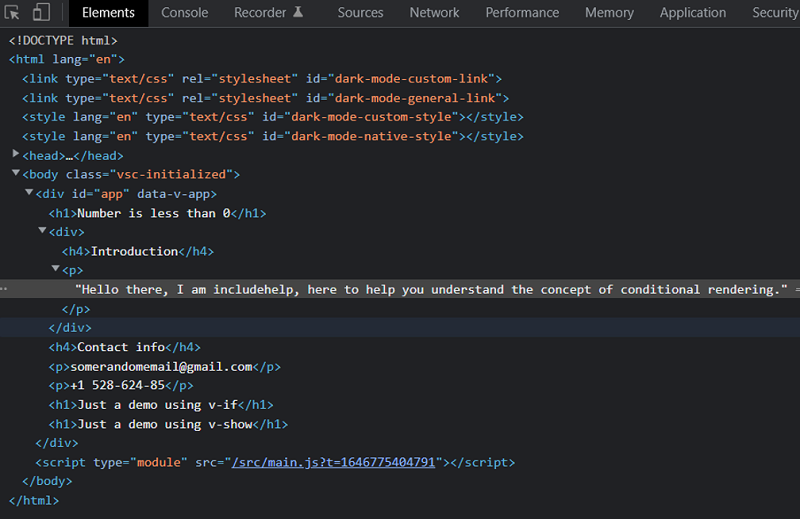• Inspecting the element shows that the v-if directive removes the element from the DOM, whereas the v-show just manipulates the display property to render the element.
• You can view this by the following shortcut Ctrl + Shift + I.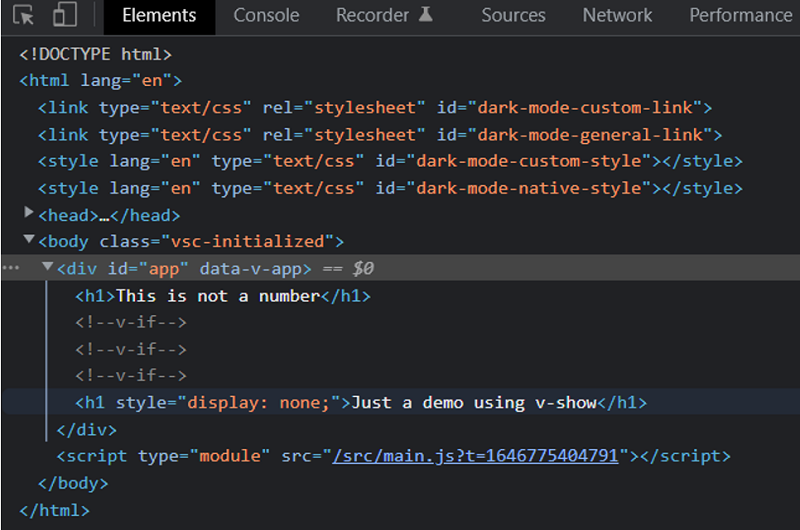Note:

Removing and adding to the DOM is an expensive operation, hence use v-if only where you have to really remove the element from the DOM, else use v-show to conditionally render.